Published: 30 May 2017

# Numerical modelling of scour around circular cylinder caused by jet flow and bed shear stress

Hyoseob Kim1
Seungho Lee2
Jungik Lee3
Hak Soo Lim4
Hee-Suk Ryoo5
1, 2, 3Kookmin University, Seoul, Republic of Korea
4Korea Institute of Ocean Science and Technology, Ansan, Republic of Korea
5Korea Electrotechnology Reasearch Institute, Changwon, Republic of Korea
Corresponding Author:
Seungho Lee
Views 71

#### Abstract

A new scour numerical model composed of two modules is proposed here. The two modules are detailed Reynolds-average Navier-Stokes flow module and sediment transport and resultant scour module. The flow module uses horizontally regular grid at the bed but partial grid concept in the vertical direction. The sediment module operates entrainment, and deposition of suspended sediment. The entrainment of sediment is computed by a new empirical equation; the major independent variables of which are the jet flow velocity and the bed shear stress. The model is applied to a laboratory scour experiment around a circular cylinder at Kookmin University, and shows satisfactory agreement with measurements.

## 1. Introduction

Modelling scour require a special grid system to allow gradual evolvement of local scour hole, which involves steep slope and sudden slope change, in contrast to modelling wide area morphologic change on coasts. There have been a few trials to overcome this problem.

First, a very fine traditional regular grid can be used. However, very fine grid involves high computation cost to resolve bed roughness. Second, a nesting or coupling technique to combine coarse and fine grids can be used to reduce computational load. However, this also requires complicated joint treatment, and does not dramatically reduce computational load. Third, a moving boundary-fitted, unstructured, or fractional grid around solid boundary can be used. However, this measure leads to heavy grid-related mid-processing, and is not free from numerical error due to non-straight grid lines, or triangular, trapezoidal, or octagonal grids.

A simple grid system, regular in plan, moving fractional in the vertical direction, is adopted here to simulate scour evolution around vertical structures.

Existing sediment entrainment or pickup rate from the bed surface has been described as a function of the bed shear stress . Our approach here is resolving short wave phase, and therefore we don’t need wave-phase-average entrainment rate, but instantaneous entrainment rate. Existing instantaneous sediment entrainment rate formulas are all empirical [3-5], and could be represented by an equation as:

1
${E}_{\tau }={C}_{1}{\left(\tau -{\tau }_{cri}\right)}^{{n}_{1}},$

where ${E}_{\tau }$ is the entrainment rate; ${C}_{1}$, ${n}_{1}$ are specific coefficients for a given sediment material, $\tau$ is the instantaneous bed shear stress, and ${\tau }_{cri}$ is the critical shear stress for sediment movement initiation. If bed sediment is non-cohesive, and its median diameter is known, the above coefficients and be found from an existing formula.

A fundamental defect of the above equation is that the equation cannot take into account the effect of local pressure enhanced by jet flow. Eq. (1) is suitable for fluid flows which are more less flat like river flows or coastal flows. It is quite obvious that sediment is eroded at the center of a circle if a jet flow hits the seabed in the normal direction, while simulation with the above equation may result in donut-type erosion, which is wrong. Flow around a vertical structure shows sheet-type jet flow along the surface of the structure which soon turns its direction, and generate horseshoe vortex.

Sumer and Fredsoe , Dixen et al.  and Sumer et al.  proposed a methodology to treat the extra entrainment due to jet flow, or horseshoe vortex in front of structures, Sumer et al. regarded the extra entrainment as a result of enhanced turbulence, and modified their bed load formula by adding a term including turbulent energy. However, the horseshoe vortex is a turbulence-average flow behavior, and thus quantifying the extra entrainment by the turbulent energy could not explain the phenomena properly. Explanation of the extra entrainment by jet flow seems to be more appropriate. Local erosion due to jet flow has been studied by experiments or numerical simulations [9-11].

## 2. Numerical model setup

The numerical model WCFLUME [12, 13] is composed of two modules, i.e. flow and sediment transport modules. The flow module solves extended governing equations and difference equations for three-dimensional domain from two-dimensional vertical domain. Model grid is basically regular rectangular, see Fig. 1. The horizontal grid is regular, see Fig. 2(a). The bed morphology is expressed as steps, the levels of which do not agree with grid border levels, see Fig. 2(b). The bed shear stress at the bed surface is computed by using the logarithmic law and the nearest available velocity above the bed, see Fig. 3.

Fig. 1Model grid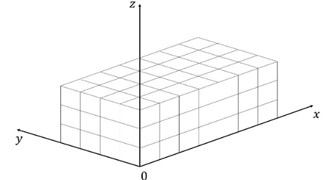Fig. 2Modification of grid in the vertical direction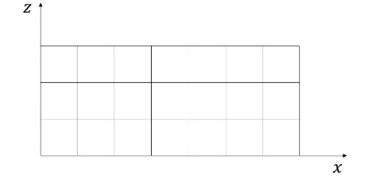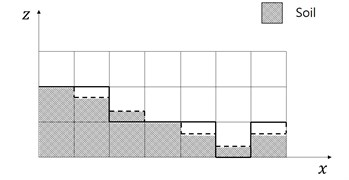The sediment transport module solves the transport and dispersion with the water column [12, 13]. The sediment entrainment into the water column is contributed by both the instantaneous bed shear stress, and the local jet. To take into account the extra-entrainment due to jet effect pressure could be used, but it is not easy to extract the jet-induced pressure increment from the total pressure field which includes both static and dynamic pressure. Alternately the vertical velocity may be used as the representative variable of the jet towards seabed. The vertical velocity just above the seabed is the most adequate to be linked to the entrainment rate instead of pressure. A weak point of using the vertical velocity above the seabed is that it is grid-size dependent. Velocity reduction gradient in the vertical direction would be the most appropriate representative variable to express the extra entrainment. In this paper the vertical velocity gradient at the seabed is adopted. Then the extra entrainment rate is expressed as:

2
${E}_{jet}={C}_{2}{\left\{\frac{\partial \left(w-{w}_{cri}\right)}{\partial z}\right\}}^{{n}_{2}},$

where ${E}_{jet}$ is the entrainment rate due to jet flow, $w$ is the vertical fluid velocity speed towards the seabed, and ${w}_{cri}$ is the critical vertical fluid velocity speed for initiation movement of sediment. A difference equation replaces the above differential equation as:

3
${E}_{jet}={C}_{2}{\left(\frac{{w}^{\mathrm{*}}-{w}_{cri}}{∆z}\right)}^{{n}_{2}},$

where ${w}^{\mathrm{*}}$ is the downward vertical fluid velocity speed at the nearest grid border from the bed. The coefficients in the above equation should be found from measurements available. Coefficients used are: ${C}_{2}=1.5$, ${C}_{2}=1.0$. Then:

4
${E={E}_{\tau }+E}_{jet}.$

Fig. 3Assumption of horizontal velocity distribution in the vertical direction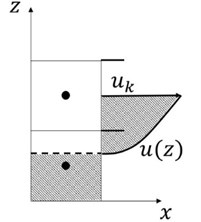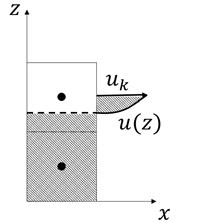## 3. Model application

The present model system WCFLUME was applied to a laboratory experiment at Kookmin University . A vertical cylinder stands in a current flume, see Fig. 4.

Model results show three-dimensional flow pattern around the cylinder, and minor surface undulation around the cylinder. Horseshoe vortex ring developed around the cylinder foot. Computed flow field at the bed in ($x-z$) domain in Fig. 5, and flow field in Fig. 6 in a $\left(x-z\right)$ section show horseshoe vortex at an intermediate stage of scour. The vortex could be expressed by vorticity, ${\mathrm{\Omega }}_{xz}$, that is:

5
${\mathrm{\Omega }}_{xz}=\frac{\partial \omega }{\partial x}-\frac{\partial u}{\partial z}.$

An interesting three-dimensional circulation behind the cylinder has been reported by Sumer et al. . Computed flow fields in the $\left(x-z\right)$ sections show this circulation pattern, see Fig. 8. Because the simulation includes free surface, computed water surface level shows the back water phenomena.

However, vorticity describes angular rotating speed of a fluid element, and thus gives positive value for even straight shear flow, see Fig. 7. If we want to extract rotationality with curvature, say curvy vorticity, we could introduce the following properties, ${\mathrm{\Omega }}_{c,\mathrm{}xz}$ and ${\mathrm{\Omega }}_{c,yz}$:

6
${\mathrm{\Omega }}_{c,\mathrm{}xz}=\left\{\begin{array}{ll}-\frac{\partial \omega }{\partial x}\left|\frac{\partial u}{\partial z}\right|,& \frac{\partial \omega }{\partial x}\bullet \frac{\partial u}{\partial x}<0,\\ 0,& \frac{\partial \omega }{\partial y}\bullet \frac{\partial u}{\partial z}\ge 0,\end{array}\right\{\mathrm{\Omega }}_{c,\mathrm{}zy}=\left\{\begin{array}{ll}-\frac{\partial \omega }{\partial y}\left|\frac{\partial v}{\partial z}\right|,& \frac{\partial \omega }{\partial x}\bullet \frac{\partial u}{\partial x}<0,\\ 0,& \frac{\partial \omega }{\partial y}\bullet \frac{\partial u}{\partial z}\ge 0.\end{array}\right\$

Fig. 4Model setup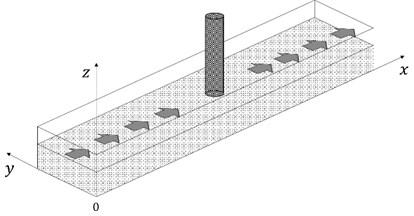Fig. 5Computed bed flow field showing horseshoe vortex in front of cylinder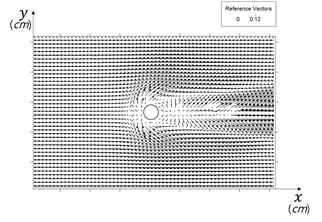Fig. 6Horseshoe vortex in front of cylinder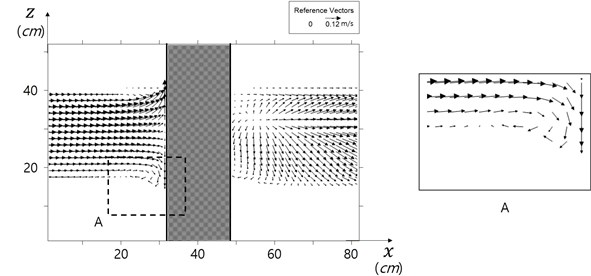Fig. 7a) Vorticity for shear flow b) curvy vorticity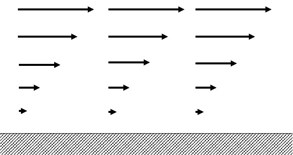a)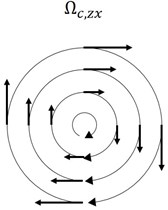b)

Computed vorticity and curvy vorticity are shown in Fig. 8. The curvy vorticity well describes the strength of rotationality, and the position of horse shoes vorticity, compared to traditional vorticity.

Computed contributions of the vertical gradient of the vertical velocity the bed shear stress and are shown in Figs. 10, and 11, and their sum is shown in Fig. 12. It is obvious that jet flow heavily contributes scour evolvement in front of the cylinder.

Computed time evolution of the scour depth at cylinder foot is shown in Fig. 13. Scour depth develops with step-shape, which may have been resulted from the partial vertical grid treatment.

Fig. 8Traditional vorticity vs curvy vorticity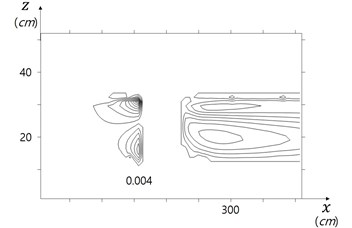a) Vorticity for shear flow (1/s)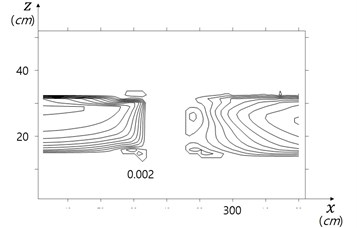b) Curvy vorticity (1/s)

Fig. 9Three-dimensional flow behind cylinder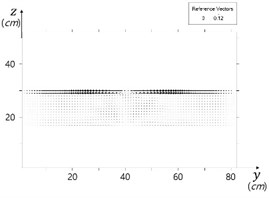a) 0.229 m behind cylinder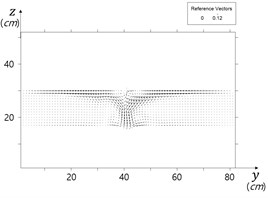b) 0.259 m behind cylinder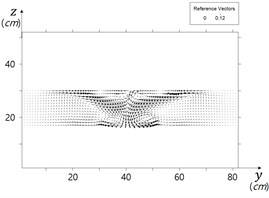c) 0.289 m behind cylinder

Fig. 10Scour depth due to jet flow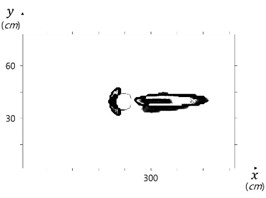Fig. 11Scour depth due to bed shear stress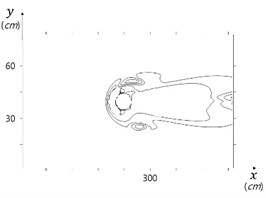Fig. 12Total scour depth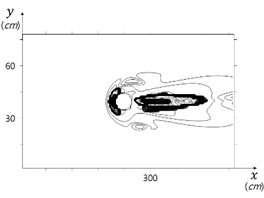Fig. 13Time evolution of scour depth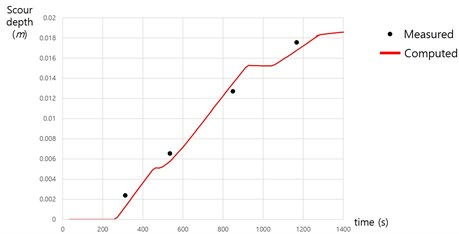## 4. Conclusions

A numerical model system, WCFLUME [12-13], was developed for simulation of local scour around coastal structures. The system uses a regular three-dimensional parallelepiped grid. The seabed level stays between grid border lines.

Sediment entrainment is expressed by a new equation which includes both existing empirical term composed of the bed shear stress, and a new term composed of the vertical gradient of the vertical velocity, which represents the jet effect.

The model system was applied to a scour around a vertical circular cylinder sandy bed. Model simulated horseshoe- vortex-induced scour hole upstream side of the cylinder, and bed-shear-stress-induced scour hole at both sides of the cylinder reasonably well. Empirical coefficients involved in simulation may further need to be assessed with more data.

07 November 2016
Accepted
08 November 2016
Published
30 May 2017
SUBJECTS
Flow induced structural vibrations
Keywords
scour
jet
bed shear stress
numerical model
curvy vorticity
Acknowledgements

This research is a part of the Project titled “Development of Coastal Erosion Control Technology”, funded by the Ministry of Oceans and Fisheries, and “Installation and Corroborative Study for Internal Power Network of Ocean Wind Power Plant”, funded by the Institute of Energy Technology Evaluation and Planning, Korea.